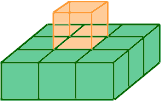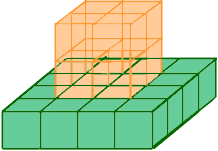Name: Katie Who is asking: Student Level: Secondary Question: This problem is about finding the number of cubes visible and hidden in a cube. In a cube that is 3x3, 19 cubes can be seen. 8 are hidden. In a cube that is 4x4, 37 cubes can be seen. 27 are hidden. In a cube that is 5x5, 61 cubes can be seen. 64 are hidden. In a cube that is 6x6, 91 cubes can be seen. 125 are hidden. The question is: Explain how you could find the number of small cubes that are visible and hidden in a cube of any size. I have no clue how to do this. Please help me figure it out. Thank You. Katie Hi Katie, A cube is three-dimensional so the cubes you mention are 3x3x3, 4x4x4, 55x5 and 6x6x6. Now I can see where the numbers come from. A 3x3x3 cube is made from 27 cubes and a 2x2x2 cube is made from 8 cubes. 27 - 8 = 19 and hence the numbers in the first row. In a cube that is 3x3x3, 19 cubes can be seen. 8 are hidden. A 4x4x4 cube is made from 64 cubes and a 3x3x3 cube is made from 27 cubes. 64 - 27 = 37 and hence the numbers in the second row. In a cube that is 4x4x4, 37 cubes can be seen. 27 are hidden. In each row the ARITHMETIC pattern is In a cube that is nxnxn, n3 - (n-1)3 cubes can be seen. (n-1)3 are hidden. What I don't see is the GEOMETRY. If you have 27 identical blocks and you start to construct a 3x3x3 cube you immediately see that there is 1 block that is hidden.If you have 64 identical blocks and you start to construct a 4x4x4 cube you immediately see that there are 8 block that is hidden.If you have n3 identical blocks and you construct an nxnxn cube there are (n-2)3 block that is hidden. Cheers, Paul and Penny Go to Math Central